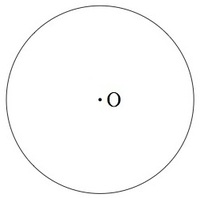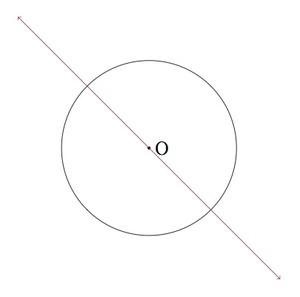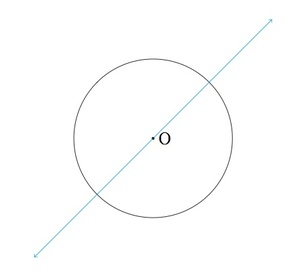# Lines of symmetry for circles

Alignments to Content Standards: 4.G.A.3

Mr. Watkins asked his students to draw a line of symmetry for a circle with center $O$ pictured below:1. Lisa drew the picture below. Is Lisa correct?3. How many lines of symmetry does a circle have? Explain.
4. Explain why each line of symmetry divides the circle in half.
5. Explain why each line of symmetry for the circle must go through the center.

## IM Commentary

A circle has an infinite number of symmetries. This contrasts with polygons such as the triangles and quadrilaterals considered in 4.G Lines of symmetry for triangles and 4.G Lines of symmetry for quadrilaterals. The circle is, in some sense, the most symmetric two dimensional figure and it is partly for this reason that it is so familiar. Coins, clock faces, wheels, the image of the full moon in the sky: these are all examples of circles which we encounter on a regular basis.

This is an instructional task that gives students a chance to reason about lines of symmetry and discover that a circle has an an infinite number of lines of symmetry. Even though the concept of an infinite number of lines is fairly abstract, fourth graders can understand infinity in an informal way. Just as there is always a fraction between any two fractions on the number line, there is always another line through the center of the circle "between" any two lines through the center of the circle. So if you identify a certain number of lines, you can argue that there is always at least one more.

• The algebraic perspective, using the equation that defines a circle, and
• The geometric perspective, using the definition of reflections in terms of perpendicular lines.

This task includes an experimental GeoGebra worksheet, with the intent that instructors might use it to more interactively demonstrate the relevant content material. The file should be considered a draft version, and feedback on it in the comment section is highly encouraged, both in terms of suggestions for improvement and for ideas on using it effectively. The file can be run via the free online application GeoGebra, or run locally if GeoGebra has been installed on a computer.

## Attached Resources

• 1060 GeoGebra File
• ## Solution

1. Lisa is correct. If we fold the circle over the line she has drawn then the parts of the circle on each side of the line match up.
2. Brad is also correct. If we fold the circle over the line he has drawn then the parts of the circle on each side of the line match up.
3. If we fold the circle over any line through the center $O$, then the parts of the circle on each side of the line will match up. One way to create such a line is to pick a point on the top half of the circle and draw the line through that point and the center $O$. Just like there are an infinite number of points on a line (if you pick any two points, there is always another one in between them) there are an infinite number of points on the top half of the circle. Each of these points can be used to draw a line of symmetry. Since there are an infinite number of lines through the center, the circle has an infinite number of lines of symmetry.

4. When the circle is folded over a line of symmetry, the parts of the circle on each side of the line match up. This means that the parts of the circle on each side of the line must have the same area. So a line of symmetry divides the circle into two parts with equal area.
5. A line of symmetry for the circle must cut the circle into two parts with equal area. Below is a picture of two lines not containing $O$:Note that in each case, for a line $L$ through the circle that does not contain the center $O$, the part of the circle on the side of $L$ that contains $O$ is larger than the part of the circle on the side of $L$ which does not contain $O$. Hence these lines cannot be lines of symmetry as any line of symmetry would cut the circle in half.